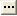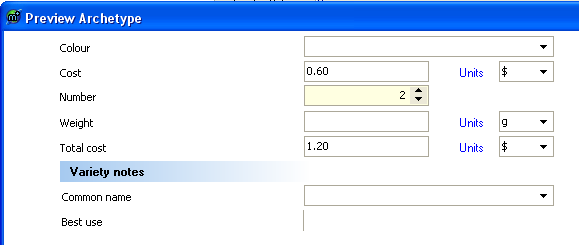GELLO code will be used to calculate the value of 'Total Cost'. It will retrieve the values from 'Number' and 'Cost' and return the result of one multiplied by the other. Click on the RHS in the pane next to IsCalculated for the 'Total Cost'. Then click onon the far right hand side of this pane, which is now a white background colour.

1. The first bit of GELLO code is prefilled and sets the context

 `Context CEN_Apple_v1::ArchetypeRoot`

2. Now let's set up a local variable to hold the value for instances of the archetype where 'Cost' is entered. Add the following code:

 `Let cost: Integer = template.Apple.Cost.value.oclAsType(PQ).value`

3. Same again for 'Number':

 `Let number:Integer = template.Apple.Number.value.oclAsType(Integer)`

4. Test this code if you like with the compile button.5. Next thing is to do the line that produces the result:

 `Let result_Real: Real = cost * number`

6. But 'Total Cost' is a Physical Quantity*, so the result needs to be returned in this form. This line of code just declares what needs to be returned by the GELLO expression:

 ```--- PQ{value = result_Real, unit = '\$'}```

 * It isn't really, but we are using this apple example to learn about such things.

7. Running in Preview Archetype:8. Don't forget to save the archetype!!